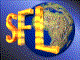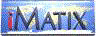| iMatix home page | << | < | > | >>SFLVersion 2.11

### encode_base64

```#include "sflmime.h"
size_t
encode_base64 (const byte *source, byte *target, size_t source_size)
```

#### Synopsis

Encodes a source buffer in Base 64 and stores the result in the target buffer. The target buffer must be at least 1/3rd longer than the amount of data in the source buffer. The base64 data consists of portable printable characters as defined in RFC 1521. Returns the number of bytes output into the target buffer.

#### Source Code - (sflmime.c)

```{
size_t
target_size = 0;                /*  Length of target buffer          */
int
nb_block;                       /*  Total number of blocks           */
byte
*p_source,                      /*  Pointer to source buffer         */
*p_target,                      /*  Pointer to target buffer         */
value;                          /*  Value of Base64 byte             */

ASSERT (source);
ASSERT (target);

if (source_size == 0)
return (0);

if (!tables_initialised)
init_conversion_tables ();

/*    Bit positions
| byte 1 | byte 2 | byte 3 |
source block   87654321 87654321 87654321         -> 3 bytes of 8 bits

| byte 1 | byte 2 | byte 3 | byte 4 |
Encoded block  876543   218765   432187   654321  -> 4 bytes of 6 bits
*/

nb_block = (int) (source_size / 3);

/*  Check if we have a partially-filled block                            */
if (nb_block * 3 != (int) source_size)
nb_block++;
target_size = (size_t) nb_block * 4;
target [target_size] = '\0';

p_source = (byte *) source;         /*  Point to start of buffers        */
p_target = target;

while (nb_block--)
{
/*  Byte 1                                                           */
value       = *p_source >> 2;
*p_target++ = base64_to_char [value];

/*  Byte 2                                                           */
value = (*p_source++ & 0x03) << 4;
if ((size_t) (p_source - source) < source_size)
value |= (*p_source & 0xF0) >> 4;
*p_target++ = base64_to_char [value];

/*  Byte 3 - pad the buffer with '=' if block not completed          */
if ((size_t) (p_source - source) < source_size)
{
value = (*p_source++ & 0x0F) << 2;
if ((size_t) (p_source - source) < source_size)
value |= (*p_source & 0xC0) >> 6;
*p_target++ = base64_to_char [value];
}
else
*p_target++ = '=';

/*  Byte 4 - pad the buffer with '=' if block not completed          */
if ((size_t) (p_source - source) < source_size)
{
value       = *p_source++ & 0x3F;
*p_target++ = base64_to_char [value];
}
else
*p_target++ = '=';
}
return (target_size);
}
```

 | << | < | > | >>Copyright © 1996-2000 iMatix Corporation